# Test Prep Plan - Take a practice test

Take this practice test to check your existing knowledge of the course material. We'll review your answers and create a Test Prep Plan for you based on your results.
How Test Prep Plans work
1
2Based on your results, we'll create a customized Test Prep Plan just for you!
3Study smarter
Study more effectively: skip concepts you already know and focus on what you still need to learn.

# Continuity of Functions Chapter Exam

Exam Instructions:

Choose your answers to the questions and click 'Next' to see the next set of questions. You can skip questions if you would like and come back to them later with the yellow "Go To First Skipped Question" button. When you have completed the practice exam, a green submit button will appear. Click it to see your results. Good luck!

### Page 1

#### Question 1 1. Consider the function below. According to the intermediate value theorem, is there a solution to f(x) = 0 for a value of x between -5 and 5?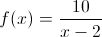#### Question 3 3. What are the regions of continuity given the following?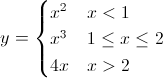#### Question 4 4. What are the regions of continuity in this graph of f(x)?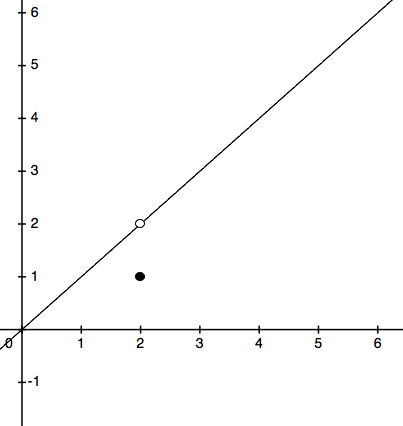#### Question 5 5. What are the regions of continuity in the graph of f(x) below?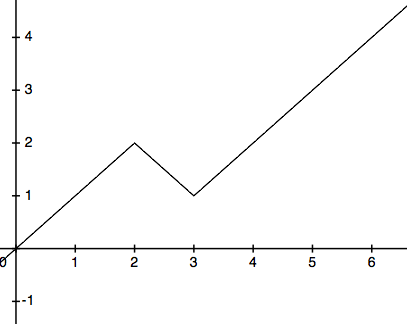### Page 2

#### Question 6 6. Consider the function f(x) below. Considering the intermediate value theorem and the vertical asymptote at x=0, is the following statement true or false? For any number C greater than 0, there is a positive value of x that satisfies the equation f(x)=C.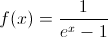#### Question 7 7. What are the regions of continuity for y defined as?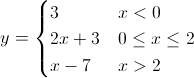### Page 3

#### Question 11 11. Given the values of the continuous function f(x) below, how many solutions will there be to f(x)=4.1?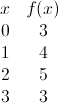#### Question 13 13. Consider the equation below. Calculate f(0), f(1) and f(2). Given that information, how many solutions are there to f(x)=-1?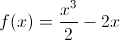#### Question 15 15. Given the function below, in what region will f(x) = 0?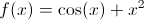### Page 4

#### Question 17 17. Given y below and the intermediate value theorem, how many times will y = 0 between x = 0 and x = π? (Hint: Work in radians since one of the parameters for x is π.)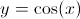#### Question 18 18. How many discontinuities are in this function?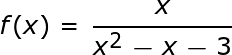### Page 5

#### Question 24 24. Which of the following continuous functions will have at least one solution to the following equation?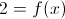#### Question 25 25. Consider the function below. According to the intermediate value theorem, is there a solution to f(x) = 4 for a value of x between 4 and 7?#### Continuity of Functions Chapter Exam Instructions

Choose your answers to the questions and click 'Next' to see the next set of questions. You can skip questions if you would like and come back to them later with the yellow "Go To First Skipped Question" button. When you have completed the practice exam, a green submit button will appear. Click it to see your results. Good luck!

Support